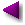Home | Mathematics | * Consumer Loan Calculator * Applied Mathematics * Calculus * Finance Calculator * Is Mathematics a Science? * Maxima * Sage * Trigonometric Relations * Unit Conversions Area of an Irregular Polygon Binomial Probability Equities Myths Graphinity Graphitude Interactive LaTeX Editor Introduction to Statistics Mandelbrot Set Peak People Polygon Calculator Polynomial Regression Data Fit Polynomial Regression Data Fit (Java version) Prime Numbers Quadratic Equation Solver Randomness Signal Processing The Mathematics of Pi The Mathematics of Population Increase The Physics Behind Stopping a Car Why PDF?Share This Page

##The Mathematics of Population Increase

-- P. LutusMessage Page --

(Updated 09.28.2015)

The equations below can be used to calculate population growth rates in a simple, easily understood way. There are better mathematical treatments for real-world applications, like the Logistic Function to describe a system with limited resources, or the many cases where the birth and death rates aren't proportional to each other, but this page should establish an accessible grounding in the basic ideas.

Basic Relationship

We will use the equation below to derive all the other forms:

(1) $\displaystyle \log\left(\frac{N}{N_{0}}\right) = r t$
Where:
• N0 (initial population) = The population at time t = 0.
• N (future population) = The population at time t.
• r (rate) = The rate of population change as a function of t (a 1% increase is expressed as 0.01).
• This variable is called the Malthusian Parameter.
• In population studies, r is usually taken to mean births minus deaths.
• t (time) = The amount of time required to produce a growth in population proportional to N/N0.

Derived Forms

Here are the forms of equation (1) in terms of each of its variables:

(2) $\displaystyle N = N_{0} e^{\left(r t\right)}$ (future population)

(3) $\displaystyle N_0 = N e^{\left(-r t\right)}$ (present population)

(4) $\displaystyle t = \frac{\log\left(\frac{N}{N_{0}}\right)}{r}$ (time)

(5) $\displaystyle r = \frac{\log\left(\frac{N}{N_{0}}\right)}{t}$ (rate)

Note that e in the above equations is the base of natural logarithms, and log(x) refers to the natural logarithm of x.

Doubling time

At the present world population growth rate of 1.1% per year (Population Growth), how long will it take to double the world's population?

The appropriate equation for this case is (4) above, with the following arguments:

$\displaystyle t = \frac{\log\left(\frac{N}{N_{0}}\right)}{r} = \frac{\log\left(\frac{2}{1}\right)}{0.011} = 63.243$ (years)

This equation shows that it will take about 63 years to double the world's population. If this prediction is borne out, there will be 14 billion people on Earth in 2074 (based on the 2011 population of 7 billion).

Rate of Increase

The base equation for this case is (2) above:

$\displaystyle N = N_{0} e^{\left(r t\right)}$

But because we want the increase per day, we will use this form:

$\displaystyle N_d = N_{0} e^{\left(r t\right)} - N_0$

For this problem we will use 7 billion (2011) for the initial population N0, 0.011 for the rate of increase r, and 1/365 for the argument of time t, since we want the rate of population increase per day (Nd). The result:

$\displaystyle N_d = N_{0} e^{\left(r t\right)} - N_0 = 210195$ (people per day)

 Home | Mathematics | * Consumer Loan Calculator * Applied Mathematics * Calculus * Finance Calculator * Is Mathematics a Science? * Maxima * Sage * Trigonometric Relations * Unit Conversions Area of an Irregular Polygon Binomial Probability Equities Myths Graphinity Graphitude Interactive LaTeX Editor Introduction to Statistics Mandelbrot Set Peak People Polygon Calculator Polynomial Regression Data Fit Polynomial Regression Data Fit (Java version) Prime Numbers Quadratic Equation Solver Randomness Signal Processing The Mathematics of Pi The Mathematics of Population Increase The Physics Behind Stopping a Car Why PDF?Share This Page